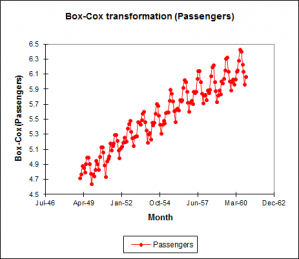# Time series transformation

Several time series transformations including Box-Cox can be accessed in Excel using the XLSTAT add-on statistical software.XLSTAT offers four different possibilities for transforming a time series Xt into Yt, (t=1,…,n):

## Box-Cox transform (fixed or optimised)

Box-Cox transformation is used to improve the normality of the time series; the Box-Cox transformation is defined by the following equation:

Yt = [ ( X2t - 1 ) / λ , (Xt > 0, λ ≠ 0 ) or (Xt ≥ 0, λ > 0 ) ; ln( Xt ), (Xt > 0, λ = 0) ]

XLSTAT accepts a fixed value of λ, or it can find the value that maximizes the likelihood of the residuals, the model being a simple linear model with the time as sole explanatory variable.

## Differencing (1-B)d(1-Bs)D

Differencing is to remove trend and seasonalities and to obtain stationarity of the time series. The difference equation writes:

Yt = (1-B)d (1-Bs)D Xt

Where d is the order of the first differencing component, s is the period of the seasonal component, D is the order of the seasonal component, and B is the lag operator defined by:

BXt = Xt-1

The values of (d, D, s) can be chosen in a trial and error process or guessed by looking at the descriptive functions (ACF, PACF). Typical values are (1,1,s), (2,1,s). s is 12 for monthly data with a yearly seasonality, 0 when there is no seasonality.

## Detrending and deseasonalizing

Detrending and deseasonalizing using the classical decomposition model which writes:

Xt = mt + st + εt

Where mt is the trend component and st the seasonal component, and εt is a N(0,1) white noise component.

XLSTAT allows fitting this model in two separate and/or successive steps:

### DETRENDING BY POLYNOMIAL REGRESSION

X t = m t + ε t = Σi=0..k aiti + εt

Where k is the polynomial degree. The ai parameters are obtained by fitting a linear model to the data. The transformed time series writes:

Y t = ε t = X t - = Σi=0..p aiti

### DESAISONALIZATION BY LINEAR MODEL

Xt = st + εt = µ + bi + εt, i = t mod p

Where p is the period. The bi parameters are obtained by fitting a linear model to the data. The transformed time series writes: Yt = εt = Xt - µ - bi

Note: there are many other possible transformations like Empirical Mode Decomposition (EMD) or Ensemble Empirical Mode Decomposition (EEMD). Some of them are available in the transformations tool of XLSTAT: see the data transformation section. Linear filters may also be applied. Moving average smoothing methods which are linear filters are available in the Smoothing tool of XLSTAT.### analyze your data with xlstat

14-day free trial

Included in

Related features